# Data Structure and Algorithms - Linked List

If arrays accommodate similar types of data types, linked lists consist of elements with different data types that are also arranged sequentially.

But how are these linked lists created?

A linked list is a collection of “nodes” connected together via links. These nodes consist of the data to be stored and a pointer to the address of the next node within the linked list. In the case of arrays, the size is limited to the definition, but in linked lists, there is no defined size. Any amount of data can be stored in it and can be deleted from it.

There are three types of linked lists −

• Singly Linked List − The nodes only point to the address of the next node in the list.

• Doubly Linked List − The nodes point to the addresses of both previous and next nodes.

• Circular Linked List − The last node in the list will point to the first node in the list. It can either be singly linked or doubly linked.

Linked list can be visualized as a chain of nodes, where every node points to the next node.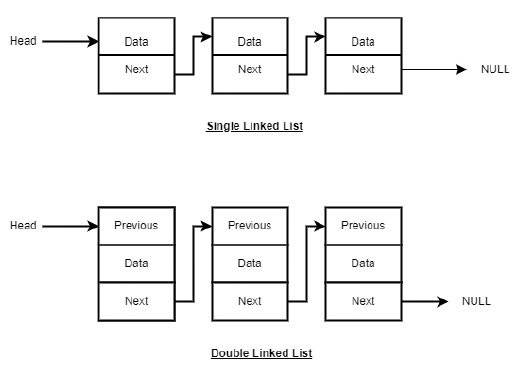As per the above illustration, following are the important points to be considered.

• Each link carries a data field(s) and a link field called next.

• Last link carries a link as null to mark the end of the list.

Following are the various types of linked list.

Singly linked lists contain two “buckets” in one node; one bucket holds the data and the other bucket holds the address of the next node of the list. Traversals can be done in one direction only as there is only a single link between two nodes of the same list.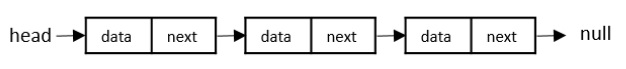Doubly Linked Lists contain three “buckets” in one node; one bucket holds the data and the other buckets hold the addresses of the previous and next nodes in the list. The list is traversed twice as the nodes in the list are connected to each other from both sides.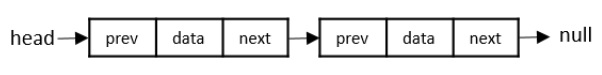Since the last node and the first node of the circular linked list are connected, the traversal in this linked list will go on forever until it is broken.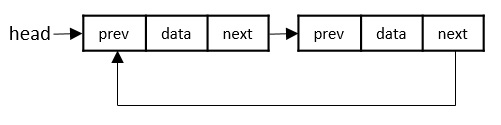## Basic Operations in the Linked Lists

The basic operations in the linked lists are insertion, deletion, searching, display, and deleting an element at a given key. These operations are performed on Singly Linked Lists as given below −

• Insertion − Adds an element at the beginning of the list.

• Deletion − Deletes an element at the beginning of the list.

• Display − Displays the complete list.

• Search − Searches an element using the given key.

• Delete − Deletes an element using the given key.

## Insertion Operation

Adding a new node in linked list is a more than one step activity. We shall learn this with diagrams here. First, create a node using the same structure and find the location where it has to be inserted.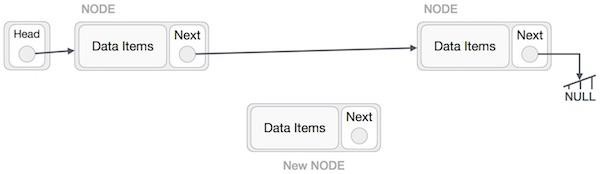Imagine that we are inserting a node B (NewNode), between A (LeftNode) and C (RightNode). Then point B.next to C −

```NewNode.next −> RightNode;
```

It should look like this −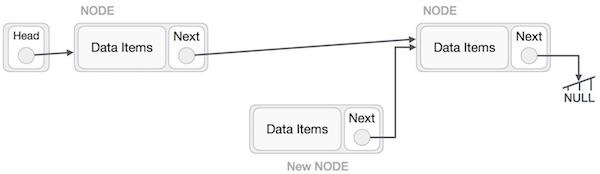Now, the next node at the left should point to the new node.

```LeftNode.next −> NewNode;
```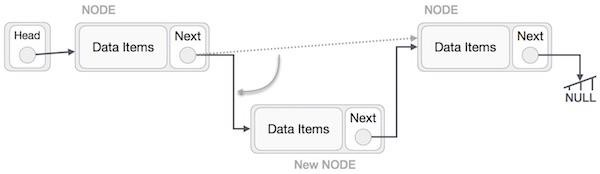This will put the new node in the middle of the two. The new list should look like this −

Insertion in linked list can be done in three different ways. They are explained as follows −

### Insertion at Beginning

In this operation, we are adding an element at the beginning of the list.

### Algorithm

```1. START
2. Create a node to store the data
3. Check if the list is empty
4. If the list is empty, add the data to the node and assign the head pointer to it.
6. END
```

### Example

Following are the implementations of this operation in various programming languages −

```#include <stdio.h>
#include <string.h>
#include <stdlib.h>
struct node {
int data;
struct node *next;
};
struct node *current = NULL;

// display the list
void printList(){
printf("\n[");

//start from the beginning
while(p != NULL) {
printf(" %d ",p->data);
p = p->next;
}
printf("]");
}

//insertion at the beginning
void insertatbegin(int data){

struct node *lk = (struct node*) malloc(sizeof(struct node));
lk->data = data;

// point it to old first node

//point first to new first node
}
void main(){
int k=0;
insertatbegin(12);
insertatbegin(22);
insertatbegin(30);
insertatbegin(44);
insertatbegin(50);

// print list
printList();
}
```

### Output

```Linked List:
[ 50  44  30  22  12 ]
```
```#include <bits/stdc++.h>
#include <string>
using namespace std;
struct node {
int data;
struct node *next;
};
struct node *current = NULL;

// display the list
void printList(){
cout << "\n[";

//start from the beginning
while(p != NULL) {
cout << " " << p->data << " ";
p = p->next;
}
cout << "]";
}

//insertion at the beginning
void insertatbegin(int data){

struct node *lk = (struct node*) malloc(sizeof(struct node));
lk->data = data;

// point it to old first node

//point first to new first node
}
int main(){
insertatbegin(12);
insertatbegin(22);
insertatbegin(30);
insertatbegin(44);
insertatbegin(50);

// print list
printList();
}
```

### Output

```Linked List:
[ 50  44  30  22  12 ]
```
```public class Linked_List {
static class node {
int data;
node next;
node (int value) {
data = value;
next = null;
}
}

// display the list
static void printList() {
System.out.print("\n[");

//start from the beginning
while(p != null) {
System.out.print(" " + p.data + " ");
p = p.next;
}
System.out.print("]");
}

//insertion at the beginning
static void insertatbegin(int data) {

node lk = new node(data);;

// point it to old first node

//point first to new first node
}
public static void main(String args[]) {
int k=0;
insertatbegin(12);
insertatbegin(22);
insertatbegin(30);
insertatbegin(44);
insertatbegin(50);
insertatbegin(33);

// print list
printList();
}
}
```

### Output

```Linked List:

[33  50  44  30  22  12 ]
```
```class Node:
def __init__(self, data=None):
self.data = data
self.next = None
class SLL:
def __init__(self):

def listprint(self):
while printval is not None:
print (printval.data)
printval = printval.next
NewNode = Node(newdata)

# Update the new nodes next val to existing node

l1 = SLL()
e2 = Node("672")
e3 = Node("63")

e2.next = e3

l1.listprint()
```

### Output

```Linked List:
122
731
672
63
```

### Insertion at Ending

In this operation, we are adding an element at the ending of the list.

### Algorithm

```1. START
2. Create a new node and assign the data
3. Find the last node
4. Point the last node to new node
5. END
```

### Example

Following are the implementations of this operation in various programming languages −

```#include <stdio.h>
#include <string.h>
#include <stdlib.h>
struct node {
int data;
struct node *next;
};
struct node *current = NULL;

// display the list
void printList(){
printf("\n[");

//start from the beginning
while(p != NULL) {
printf(" %d ",p->data);
p = p->next;
}
printf("]");
}

//insertion at the beginning
void insertatbegin(int data){

struct node *lk = (struct node*) malloc(sizeof(struct node));
lk->data = data;

// point it to old first node

//point first to new first node
}
void insertatend(int data){

struct node *lk = (struct node*) malloc(sizeof(struct node));
lk->data = data;

// point it to old first node

//point first to new first node
}
void main(){
int k=0;
insertatbegin(12);
insertatend(22);
insertatend(30);
insertatend(44);
insertatend(50);

// print list
printList();
}
```

### Output

```Linked List:
[ 12 22 30 44 50 ]
```
```#include <bits/stdc++.h>
#include <string>
using namespace std;
struct node {
int data;
struct node *next;
};
struct node *current = NULL;

// display the list
void printList(){
cout << "\n[";

//start from the beginning
while(p != NULL) {
cout << " " << p->data << " ";
p = p->next;
}
cout << "]";
}

//insertion at the beginning
void insertatbegin(int data){

struct node *lk = (struct node*) malloc(sizeof(struct node));
lk->data = data;

// point it to old first node

//point first to new first node
}
void insertatend(int data){

struct node *lk = (struct node*) malloc(sizeof(struct node));
lk->data = data;

// point it to old first node

//point first to new first node
}
int main(){
insertatbegin(12);
insertatend(22);
insertatbegin(30);
insertatend(44);
insertatbegin(50);

// print list
printList();
}
```

### Output

```Linked List:
[ 50  30  12  22  44 ]
```
```public class Linked_List {
static class node {
int data;
node next;
node (int value) {
data = value;
next = null;
}
}

// display the list
static void printList() {
System.out.print("\n[");

//start from the beginning
while(p != null) {
System.out.print(" " + p.data + " ");
p = p.next;
}
System.out.print("]");
}

//insertion at the beginning
static void insertatbegin(int data) {

node lk = new node(data);;

// point it to old first node

//point first to new first node
}
static void insertatend(int data) {

node lk = new node(data);

// point it to old first node

//point first to new first node
}
public static void main(String args[]) {
int k=0;
insertatbegin(12);
insertatbegin(22);
insertatbegin(30);
insertatend(44);
insertatend(50);
insertatend(33);

// print list
printList();
}
}
```

### Output

```Linked List:
[ 30  22  12  44 50  33 ]
```
```class Node:
def __init__(self, data=None):
self.data = data
self.next = None
class LL:
def __init__(self):
def listprint(self):
while val is not None:
print(val.data)
val = val.next

l1 = LL()
l2 = Node("12")
l3 = Node("7")
l4 = Node("14")
l5 = Node("61")

# Linking the first Node to second node

# Linking the second Node to third node
l2.next = l3
l3.next = l4
l4.next = l5
l1.listprint()
```

### Output

```Linked List:
23
12
7
14
61
```

### Insertion at a Given Position

In this operation, we are adding an element at any position within the list.

### Algorithm

```1. START
2. Create a new node and assign data to it
3. Iterate until the node at position is found
4. Point first to new first node
5. END
```

### Example

Following are the implementations of this operation in various programming languages −

```#include <stdio.h>
#include <string.h>
#include <stdlib.h>
struct node {
int data;
struct node *next;
};
struct node *current = NULL;

// display the list
void printList(){
printf("\n[");

//start from the beginning
while(p != NULL) {
printf(" %d ",p->data);
p = p->next;
}
printf("]");
}

//insertion at the beginning
void insertatbegin(int data){

struct node *lk = (struct node*) malloc(sizeof(struct node));
lk->data = data;

// point it to old first node

//point first to new first node
}
void insertafternode(struct node *list, int data){
struct node *lk = (struct node*) malloc(sizeof(struct node));
lk->data = data;
lk->next = list->next;
list->next = lk;
}
void main(){
int k=0;
insertatbegin(12);
insertatbegin(22);

// print list
printList();
}
```

### Output

```Linked List:
[ 22 12 30 ]
```
```#include <bits/stdc++.h>
#include <string>
using namespace std;
struct node {
int data;
struct node *next;
};
struct node *current = NULL;

// display the list
void printList(){
cout << "\n[";

//start from the beginning
while(p != NULL) {
cout << " " << p->data << " ";
p = p->next;
}
cout << "]";
}

//insertion at the beginning
void insertatbegin(int data){

struct node *lk = (struct node*) malloc(sizeof(struct node));
lk->data = data;

// point it to old first node

//point first to new first node
}
void insertafternode(struct node *list, int data){
struct node *lk = (struct node*) malloc(sizeof(struct node));
lk->data = data;
lk->next = list->next;
list->next = lk;
}
int main(){
insertatbegin(12);
insertatbegin(22);
insertatbegin(30);

// print list
printList();
}
```

### Output

```Linked List:
[ 30  22  44  50  12 ]
```
```public class Linked_List {
static class node {
int data;
node next;
node (int value) {
data = value;
next = null;
}
}

// display the list
static void printList() {
System.out.print("\n[");

//start from the beginning
while(p != null) {
System.out.print(" " + p.data + " ");
p = p.next;
}
System.out.print("]");
}

//insertion at the beginning
static void insertatbegin(int data) {

node lk = new node(data);;

// point it to old first node

//point first to new first node
}
static void insertafternode(node list, int data) {
node lk = new node(data);
lk.next = list.next;
list.next = lk;
}
public static void main(String args[]) {
int k=0;
insertatbegin(12);
insertatbegin(22);
insertatbegin(30);
insertatbegin(44);

// print list
printList();
}
}
```

### Output

```Linked List:

[44  30  50  33  22  12 ]
```
```class Node:
def __init__(self, data=None):
self.data = data
self.next = None

class SLL:
def __init__(self):

def listprint(self):
while printval is not None:
print (printval.data)
printval = printval.next

def InsertAtPos(self,nodeatpos,newdata):
if nodeatpos is None:
print("The mentioned node is absent")
return
NewNode = Node(newdata)
NewNode.next = nodeatpos.next
nodeatpos.next = NewNode

l1 = SLL()
e2 = Node("672")
e3 = Node("63")

e2.next = e3

l1.listprint()
```

### Output

```Linked List:
731
672
122
63
```

## Deletion Operation

Deletion is also a more than one step process. We shall learn with pictorial representation. First, locate the target node to be removed, by using searching algorithms.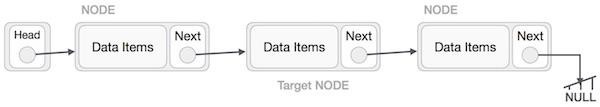The left (previous) node of the target node now should point to the next node of the target node −

```LeftNode.next −> TargetNode.next;
```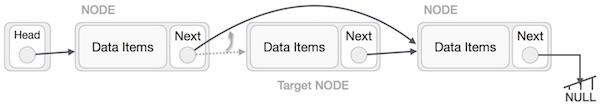This will remove the link that was pointing to the target node. Now, using the following code, we will remove what the target node is pointing at.

```TargetNode.next −> NULL;
```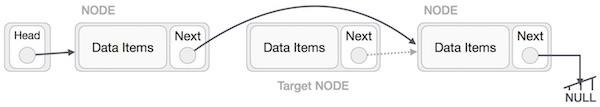We need to use the deleted node. We can keep that in memory otherwise we can simply deallocate memory and wipe off the target node completely.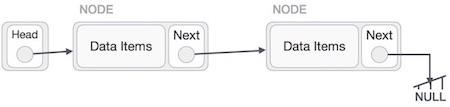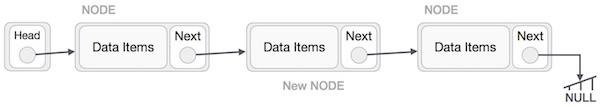Similar steps should be taken if the node is being inserted at the beginning of the list. While inserting it at the end, the second last node of the list should point to the new node and the new node will point to NULL.

Deletion in linked lists is also performed in three different ways. They are as follows −

### Deletion at Beginning

In this deletion operation of the linked, we are deleting an element from the beginning of the list. For this, we point the head to the second node.

### Algorithm

```1. START
2. Assign the head pointer to the next node in the list
3. END
```

### Example

Following are the implementations of this operation in various programming languages −

```#include <stdio.h>
#include <string.h>
#include <stdlib.h>
struct node {
int data;
struct node *next;
};
struct node *current = NULL;

// display the list
void printList(){
printf("\n[");

//start from the beginning
while(p != NULL) {
printf(" %d ",p->data);
p = p->next;
}
printf("]");
}

//insertion at the beginning
void insertatbegin(int data){

struct node *lk = (struct node*) malloc(sizeof(struct node));
lk->data = data;

// point it to old first node

//point first to new first node
}
void deleteatbegin(){
}
void main(){
int k=0;
insertatbegin(12);
insertatbegin(22);
insertatbegin(30);
insertatbegin(40);
insertatbegin(55);

// print list
printList();
deleteatbegin();

// print list
printList();
}
```

### Output

```Linked List:
[ 55  40  30  22  12 ]
[ 40  30  22  12 ]
```
```#include <bits/stdc++.h>
#include <string>
using namespace std;
struct node {
int data;
struct node *next;
};
struct node *current = NULL;

// display the list
void printList(){
cout << "\n[";

//start from the beginning
while(p != NULL) {
cout << " " << p->data << " ";
p = p->next;
}
cout << "]";
}

//insertion at the beginning
void insertatbegin(int data){

struct node *lk = (struct node*) malloc(sizeof(struct node));
lk->data = data;

// point it to old first node

//point first to new first node
}
void deleteatbegin(){
}
int main(){
insertatbegin(12);
insertatbegin(22);
insertatbegin(30);
insertatbegin(44);
insertatbegin(50);

// print list
printList();
deleteatbegin();
cout << "Linked List after deletion: ";
printList();
}
```

### Output

```Linked List:
[ 50  44  30  22  12 ]
[ 44  30  22  12 ]
```
```
static class node {
int data;
node next;
node (int value) {
data = value;
next = null;
}
}

// display the list
static void printList() {
System.out.print("\n[");

//start from the beginning
while(p != null) {
System.out.print(" " + p.data + " ");
p = p.next;
}
System.out.print("]");
}

//insertion at the beginning
static void insertatbegin(int data) {

node lk = new node(data);;

// point it to old first node

//point first to new first node
}
static void deleteatbegin() {
}
public static void main(String args[]) {
int k=0;
insertatbegin(12);
insertatbegin(22);
insertatbegin(30);
insertatbegin(44);
insertatbegin(50);
insertatbegin(33);

// print list
printList();
deleteatbegin();

// print list
printList();
}
}
```

### Output

```Linked List:
[ 33  50  44  30  22  12 ]

[50  44  30  22  12 ]
```

### Deletion at Ending

In this deletion operation of the linked, we are deleting an element from the ending of the list.

### Algorithm

```1. START
2. Iterate until you find the second last element in the list.
3. Assign NULL to the second last element in the list.
4. END
```

### Example

Following are the implementations of this operation in various programming languages −

```#include <stdio.h>
#include <string.h>
#include <stdlib.h>
struct node {
int data;
struct node *next;
};
struct node *current = NULL;

// display the list
void printList(){
printf("\n[");

//start from the beginning
while(p != NULL) {
printf(" %d ",p->data);
p = p->next;
}
printf("]");
}

//insertion at the beginning
void insertatbegin(int data){

struct node *lk = (struct node*) malloc(sizeof(struct node));
lk->data = data;

// point it to old first node

//point first to new first node
}
void deleteatend(){
}
void main(){
int k=0;
insertatbegin(12);
insertatbegin(22);
insertatbegin(30);
insertatbegin(40);
insertatbegin(55);

// print list
printList();
deleteatend();

// print list
printList();
}
```

### Output

```Linked List:
[ 55  40  30  22  12 ]
[ 55  40  30  22 ]
```
```#include <bits/stdc++.h>
#include <string>
using namespace std;
struct node {
int data;
struct node *next;
};
struct node *current = NULL;

// Displaying the list
void printList(){
while(p != NULL) {
cout << " " << p->data << " ";
p = p->next;
}
}

// Insertion at the beginning
void insertatbegin(int data){

struct node *lk = (struct node*) malloc(sizeof(struct node));
lk->data = data;

// point it to old first node

//point first to new first node
}
void deleteatend(){
}
int main(){
insertatbegin(12);
insertatbegin(22);
insertatbegin(30);
insertatbegin(44);
insertatbegin(50);

// print list
printList();
deleteatend();
cout << "\nLinked List after deletion: ";
printList();
}
```

### Output

```Linked List:  50  44  30  22  12
Linked List after deletion:  50  44  30  22
```
```public class Linked_List {
static class node {
int data;
node next;
node (int value) {
data = value;
next = null;
}
}

// display the list
static void printList() {
System.out.print("\n[");

//start from the beginning
while(p != null) {
System.out.print(" " + p.data + " ");
p = p.next;
}
System.out.print("]");
}

//insertion at the beginning
static void insertatbegin(int data) {

node lk = new node(data);;

// point it to old first node

//point first to new first node
}
static void deleteatend() {
}
public static void main(String args[]) {
int k=0;
insertatbegin(12);
insertatbegin(22);
insertatbegin(30);
insertatbegin(44);
insertatbegin(50);
insertatbegin(33);

// print list
printList();

//deleteatbegin();
deleteatend();

// print list
printList();
}
}
```

### Output

```Linked List:
[ 33  50  44  30  22  12 ]

[ 33  50  44  30  22 ]
```

### Deletion at a Given Position

In this deletion operation of the linked, we are deleting an element at any position of the list.

### Algorithm

```1. START
2. Iterate until find the current node at position in the list
3. Assign the adjacent node of current node in the list to its previous node.
4. END
```

### Example

Following are the implementations of this operation in various programming languages −

```#include <stdio.h>
#include <string.h>
#include <stdlib.h>
struct node {
int data;
struct node *next;
};
struct node *current = NULL;

// display the list
void printList(){
printf("\n[");

//start from the beginning
while(p != NULL) {
printf(" %d ",p->data);
p = p->next;
}
printf("]");
}

//insertion at the beginning
void insertatbegin(int data){

struct node *lk = (struct node*) malloc(sizeof(struct node));
lk->data = data;

// point it to old first node

//point first to new first node
}
void deletenode(int key){
struct node *temp = head, *prev;
if (temp != NULL && temp->data == key) {
return;
}

// Find the key to be deleted
while (temp != NULL && temp->data != key) {
prev = temp;
temp = temp->next;
}

// If the key is not present
if (temp == NULL) return;

// Remove the node
prev->next = temp->next;
}
void main(){
int k=0;
insertatbegin(12);
insertatbegin(22);
insertatbegin(30);
insertatbegin(40);
insertatbegin(55);

// print list
printList();
deletenode(30);

// print list
printList();
}
```

### Output

```Linked List:
[ 55  40  30  22  12 ]
[ 55  40  22  12 ]
```
```#include <bits/stdc++.h>
#include <string>
using namespace std;
struct node {
int data;
struct node *next;
};
struct node *current = NULL;

// display the list
void printList(){
cout << "\n[";

//start from the beginning
while(p != NULL) {
cout << " " << p->data << " ";
p = p->next;
}
cout << "]";
}

//insertion at the beginning
void insertatbegin(int data){

struct node *lk = (struct node*) malloc(sizeof(struct node));
lk->data = data;

// point it to old first node

//point first to new first node
}
void deletenode(int key){
struct node *temp = head, *prev;
if (temp != NULL && temp->data == key) {
return;
}

// Find the key to be deleted
while (temp != NULL && temp->data != key) {
prev = temp;
temp = temp->next;
}

// If the key is not present
if (temp == NULL) return;

// Remove the node
prev->next = temp->next;
}
int main(){
insertatbegin(12);
insertatbegin(22);
insertatbegin(30);
insertatbegin(44);
insertatbegin(50);

// print list
printList();
deletenode(30);
cout << "Linked List after deletion: ";
printList();
}
```

### Output

```Linked List:
[ 50  44  30  22  12 ]Linked List after deletion:
[ 50  44  22  12 ]
```
```public class Linked_List {
static class node {
int data;
node next;
node (int value) {
data = value;
next = null;
}
}

// display the list
static void printList() {
System.out.print("\n[");

//start from the beginning
while(p != null) {
System.out.print(" " + p.data + " ");
p = p.next;
}
System.out.print("]");
}

//insertion at the beginning
static void insertatbegin(int data) {

node lk = new node(data);;

// point it to old first node

//point first to new first node
}
static void deletenode(int key) {
node prev = null;
if (temp != null && temp.data == key) {
return;
}

// Find the key to be deleted
while (temp != null && temp.data != key) {
prev = temp;
temp = temp.next;
}

// If the key is not present
if (temp == null) return;

// Remove the node
prev.next = temp.next;
}
public static void main(String args[]) {
int k=0;
insertatbegin(12);
insertatbegin(22);
insertatbegin(30);
insertatbegin(44);
insertatbegin(50);
insertatbegin(33);

// print list
printList();

//deleteatbegin();
//deleteatend();
deletenode(12);

// print list
printList();
}
}
```

### Output

```Linked List:

[ 33  50  44  30  22  12 ]

[ 33  50  44  30  22 ]
```

## Reverse Operation

This operation is a thorough one. We need to make the last node to be pointed by the head node and reverse the whole linked list.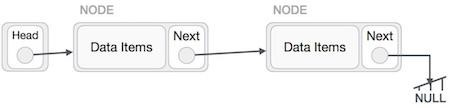First, we traverse to the end of the list. It should be pointing to NULL. Now, we shall make it point to its previous node −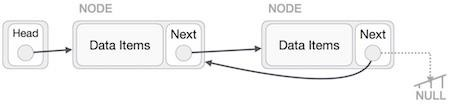We have to make sure that the last node is not the last node. So we'll have some temp node, which looks like the head node pointing to the last node. Now, we shall make all left side nodes point to their previous nodes one by one.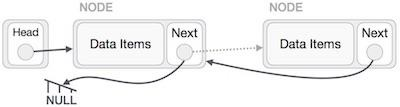Except the node (first node) pointed by the head node, all nodes should point to their predecessor, making them their new successor. The first node will point to NULL.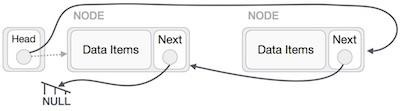We'll make the head node point to the new first node by using the temp node.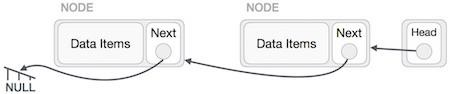### Algorithm

Step by step process to reverse a linked list is as follows −

```1 START
2. We use three pointers to perform the reversing: prev, next, head.
3. Point the current node to head and assign its next value to the prev node.
4. Iteratively repeat the step 3 for all the nodes in the list.
5. Assign head to the prev node.
```

### Example

Following are the implementations of this operation in various programming languages −

```#include <stdio.h>
#include <string.h>
#include <stdlib.h>
struct node {
int data;
struct node *next;
};
struct node *current = NULL;

// display the list
void printList(){
printf("\n[");

//start from the beginning
while(p != NULL) {
printf(" %d ",p->data);
p = p->next;
}
printf("]");
}

//insertion at the beginning
void insertatbegin(int data){

struct node *lk = (struct node*) malloc(sizeof(struct node));
lk->data = data;

// point it to old first node

//point first to new first node
}
struct node *prev = NULL, *cur=*head, *tmp;
while(cur!= NULL) {
tmp = cur->next;
cur->next = prev;
prev = cur;
cur = tmp;
}
}
void main(){
int k=0;
insertatbegin(12);
insertatbegin(22);
insertatbegin(30);
insertatbegin(40);
insertatbegin(55);

// print list
printList();
printList();
}
```

### Output

```Linked List:
[ 55  40  30  22  12 ]
[ 12  22  30  40  55 ]
```
```#include <bits/stdc++.h>
struct node {
int data;
struct node *next;
};
struct node *current = NULL;

// display the list
void printList(){
printf("\n[");

//start from the beginning
while(p != NULL) {
printf(" %d ",p->data);
p = p->next;
}
printf("]");
}

//insertion at the beginning
void insertatbegin(int data){

struct node *lk = (struct node*) malloc(sizeof(struct node));
lk->data = data;

// point it to old first node

//point first to new first node
}
struct node *prev = NULL, *cur=*head, *tmp;
while(cur!= NULL) {
tmp = cur->next;
cur->next = prev;
prev = cur;
cur = tmp;
}
}
int main(){
int k=0;
insertatbegin(12);
insertatbegin(22);
insertatbegin(30);
insertatbegin(40);
insertatbegin(55);

// print list
printList();
printList();
return 0;
}
```

### Output

```Linked List:
[ 55  40  30  22  12 ]
[ 12  22  30  40  55 ]
```
```public class Linked_List {
static class Node {
int data;
Node next;
Node (int value) {
data = value;
next = null;
}
}

// display the list
static void printList(Node node) {
System.out.print("\n[");

//start from the beginning
while(node != null) {
System.out.print(" " + node.data + " ");
node = node.next;
}
System.out.print("]");
}
Node prev = null;
Node temp = null;
while (cur != null) {
temp = cur.next;
cur.next = prev;
prev = cur;
cur = temp;
}
}
public static void main(String args[]) {

// print list
}
}
```

### Output

```Linked List:
[ 33  50  44  22  12 ]

[ 12 22  44  50  33 ]
```
```class Node:
def __init__(self, data=None):
self.data = data
self.next = None

class SLL:
def __init__(self):

def listprint(self):
while printval is not None:
print (printval.data)
printval = printval.next
def reverse(self):
prev = None
while(curr is not None):
next = curr.next
curr.next = prev
prev = curr
curr = next

l1 = SLL()
e2 = Node("672")
e3 = Node("63")

e2.next = e3

l1.listprint()
l1.reverse()
print("After reversing: ")
l1.listprint()
```

### Output

```Linked List:
731
672
63
After reversing:
63
672
731
```

## Search Operation

Searching for an element in the list using a key element. This operation is done in the same way as array search; comparing every element in the list with the key element given.

### Algorithm

```1 START
2 If the list is not empty, iteratively check if the list contains the key
3 If the key element is not present in the list, unsuccessful search
4 END
```

### Example

Following are the implementations of this operation in various programming languages −

```#include <stdio.h>
#include <string.h>
#include <stdlib.h>
struct node {
int data;
struct node *next;
};
struct node *current = NULL;

// display the list
void printList(){
printf("\n[");

//start from the beginning
while(p != NULL) {
printf(" %d ",p->data);
p = p->next;
}
printf("]");
}

//insertion at the beginning
void insertatbegin(int data){

struct node *lk = (struct node*) malloc(sizeof(struct node));
lk->data = data;

// point it to old first node

//point first to new first node
}
int searchlist(int key){
while(temp != NULL) {
if (temp->data == key) {
return 1;
}
temp=temp->next;
}
return 0;
}
void main(){
int k=0;
insertatbegin(12);
insertatbegin(22);
insertatbegin(30);
insertatbegin(40);
insertatbegin(55);

// print list
printList();
k = searchlist(30);
if (k == 1)
printf("\nElement is found");
else
printf("\nElement is not present in the list");
}
```

### Output

```Linked List:
[ 55  40  30  22  12 ]
Element is found
```
```#include <bits/stdc++.h>
#include <string>
using namespace std;
struct node {
int data;
struct node *next;
};
struct node *current = NULL;

// display the list
void printList(){
cout << "\n[";

//start from the beginning
while(p != NULL) {
cout << " " << p->data << " ";
p = p->next;
}
cout << "]";
}

//insertion at the beginning
void insertatbegin(int data){

struct node *lk = (struct node*) malloc(sizeof(struct node));
lk->data = data;

// point it to old first node

//point first to new first node
}
int searchlist(int key){
while(temp != NULL) {
if (temp->data == key) {
return 1;
}
temp=temp->next;
}
return 0;
}
int main(){
int k = 0;
insertatbegin(12);
insertatbegin(22);
insertatbegin(30);
insertatbegin(44);
insertatbegin(50);

// print list
printList();
k = searchlist(16);
if (k == 1)
cout << "\nElement is found";
else
cout << "\nElement is not present in the list";
}
```

### Output

```Linked List:
[ 50  44  30  22  12 ]
Element is not present in the list
```
```public class Linked_List {
static class node {
int data;
node next;
node (int value) {
data = value;
next = null;
}
}

// display the list
static void printList() {
System.out.print("\n[");

//start from the beginning
while(p != null) {
System.out.print(" " + p.data + " ");
p = p.next;
}
System.out.print("]");
}

//insertion at the beginning
static void insertatbegin(int data) {

node lk = new node(data);;

// point it to old first node

//point first to new first node
}
static int searchlist(int key) {
while(temp != null) {
if (temp.data == key) {
return 1;
}
temp=temp.next;
}
return 0;
}
public static void main(String args[]) {
int k=0;
insertatbegin(12);
insertatbegin(22);
insertatbegin(30);
insertatbegin(44);
insertatbegin(50);
insertatbegin(33);

// print list
printList();
k = searchlist(44);
if (k == 1)
System.out.println("\nElement is found");
else
System.out.println("\nElement is not present in the list");
}
}
```

### Output

```Linked List:

[33  50  44  30  22  12 ]
Element is found
```
```class Node:
def __init__(self, data=None):
self.data = data
self.next = None

class SLL:
def __init__(self):
def search(self, x):
count = 0

# loop till current not equal to None
while current != None:
if current.data == x:
print("data found")
count = count + 1
current = current.next
if count == 0:

l1 = SLL()
e2 = Node("672")
e3 = Node("63")

e2.next = e3

l1.search("63")
```

### Output

```data found
```

## Traversal Operation

The traversal operation walks through all the elements of the list in an order and displays the elements in that order.

### Algorithm

```1. START
2. While the list is not empty and did not reach the end of the list, print the data in each node
3. END
```

### Example

Following are the implementations of this operation in various programming languages −

```#include <stdio.h>
#include <string.h>
#include <stdlib.h>
struct node {
int data;
struct node *next;
};
struct node *current = NULL;

// display the list
void printList(){
printf("\n[");

//start from the beginning
while(p != NULL) {
printf(" %d ",p->data);
p = p->next;
}
printf("]");
}

//insertion at the beginning
void insertatbegin(int data){

struct node *lk = (struct node*) malloc(sizeof(struct node));
lk->data = data;

// point it to old first node

//point first to new first node
}
void main(){
int k=0;
insertatbegin(12);
insertatbegin(22);
insertatbegin(30);

// print list
printList();
}
```

### Output

```Linked List:
[ 30  22  12 ]
```
```#include <bits/stdc++.h>
#include <string>
using namespace std;
struct node {
int data;
struct node *next;
};
struct node *current = NULL;

// Displaying the list
void printList(){
while(p != NULL) {
cout << " " << p->data << " ";
p = p->next;
}
}

// Insertion at the beginning
void insertatbegin(int data){

struct node *lk = (struct node*) malloc(sizeof(struct node));
lk->data = data;

// point it to old first node

//point first to new first node
}
int main(){
insertatbegin(12);
insertatbegin(22);
insertatbegin(30);
insertatbegin(44);
insertatbegin(50);

// print list
printList();
}
```

### Output

```Linked List:  50  44  30  22  12
```
```public class Linked_List {
static class node {
int data;
node next;
node (int value) {
data = value;
next = null;
}
}

// display the list
static void printList() {
System.out.print("\n[");

//start from the beginning
while(p != null) {
System.out.print(" " + p.data + " ");
p = p.next;
}
System.out.print("]");
}

//insertion at the beginning
static void insertatbegin(int data) {

node lk = new node(data);;

// point it to old first node

//point first to new first node
}
public static void main(String args[]) {
int k=0;
insertatbegin(12);
insertatbegin(22);
insertatbegin(30);
insertatbegin(44);
insertatbegin(50);
insertatbegin(33);

// print list
printList();
}
}
```

### Output

```Linked List:
[ 33  50  44  30  22  12 ]
```
```class Node:
def __init__(self, data=None):
self.data = data
self.next = None
class SLL:
def __init__(self):

def listprint(self):
while printval is not None:
print (printval.data)
printval = printval.next

l1 = SLL()
e2 = Node("672")
e3 = Node("63")

e2.next = e3

l1.listprint()
```

### Output

```Linked List:
731
672
63
```